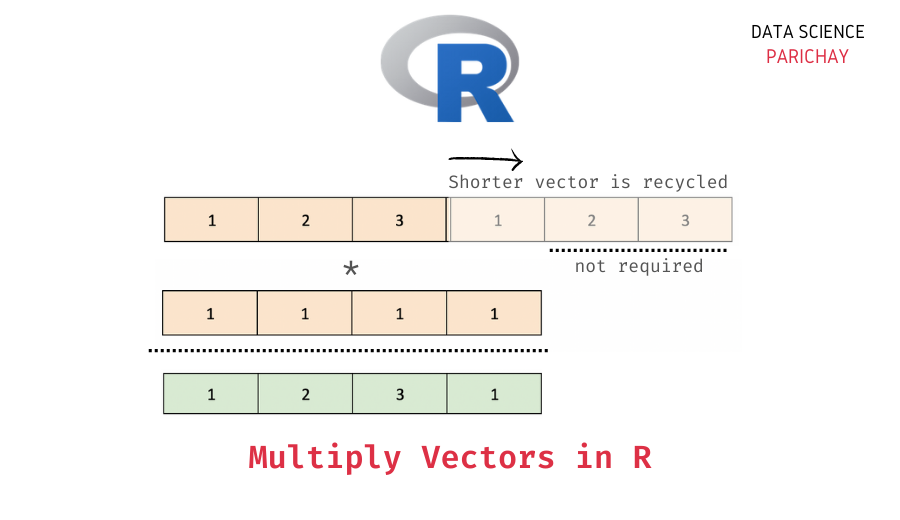# R – Multiply Two Vectors Elementwise

In this tutorial, we will look at how to multiply two vectors (element-wise) in the R programming language with the help of some examples.

## Elementwise Vector Multiplication in R

You can use the `*` operator to multiply two vectors in R. Arithmetic operations on vectors are computed element-wise. That is when you multiply two vectors, the corresponding elements are multiplied together.

If the vectors are of the same length, corresponding elements (elements with the same index) are multiplied together. The following image illustrates this.

If the vectors are of different lengths, the shorter vector will be recycled to match the length of the longer vector after which the element-wise multiplication is computed. The following image shows how this is done.

## Examples

Let’s look at some examples of multiplying two vectors (element-wise) in R.

### Multiply two numeric vectors of the same length in R

First, let’s multiply two numeric vectors having the same length using the `*` operator.

📚 Data Science Programs By Skill Level

Introductory

Intermediate ⭐⭐⭐

🔎 Find Data Science Programs 👨‍💻 111,889 already enrolled

Disclaimer: Data Science Parichay is reader supported. When you purchase a course through a link on this site, we may earn a small commission at no additional cost to you. Earned commissions help support this website and its team of writers.

```# create two vectors
v1 <- c(1, 2, 3)
v2 <- c(0, 1, 2)
# multiply vectors v1 and v1
v <- v1 * v2
# display the resulting vector
print(v)```

Output:

` 0 2 6`

Here, we multiply two vectors of length three. The resulting vector is also of length three with each element resulting from the corresponding elementwise multiplication of vectors v1 and v2.

### Multiply two numeric vectors with different lengths in R

Let’s now multiply two numeric vectors having different lengths.

```# create two vectors
v1 <- c(1, 2, 3)
v2 <- c(1, 1, 1, 1, 1)
# multiply vectors v1 and v1
v <- v1 * v2
# display the resulting vector
print(v)```

Output:

```Warning message in v1 * v2:
“longer object length is not a multiple of shorter object length”
 1 2 3 1 2```

Here, we multiply vector v1 (of length 3) with vector v2 (of length 5). You can see that the resulting vector is of length 5.

The shorter vector is recycled in order to compute the element-wise product with the longer vector. The values 1 and 2 are recycled to the index four and five respectively. Note that we get a warning that the longer vector’s length is not a multiple of the shorter vector’s length. This is why the shorter vector was not completely recycled, only the first two values were recycled to match the length of the longer vector.

Let’s look at another example. Here, the longer vector’s length is a multiple of the shorter vector’s length.

```# create two vectors
v1 <- c(1, 2, 3)
v2 <- c(1, 1, 1, 1, 1, 1)
# multiply vectors v1 and v1
v <- v1 * v2
# display the resulting vector
print(v)```

Output:

` 1 2 3 1 2 3`

We get the resulting vector of length six (same as the length of the longer vector). Also, note that we don’t get any warnings here because the shorter vector is recycled once completely to compute the multiplication with the longer vector.

You might also be interested in –

•